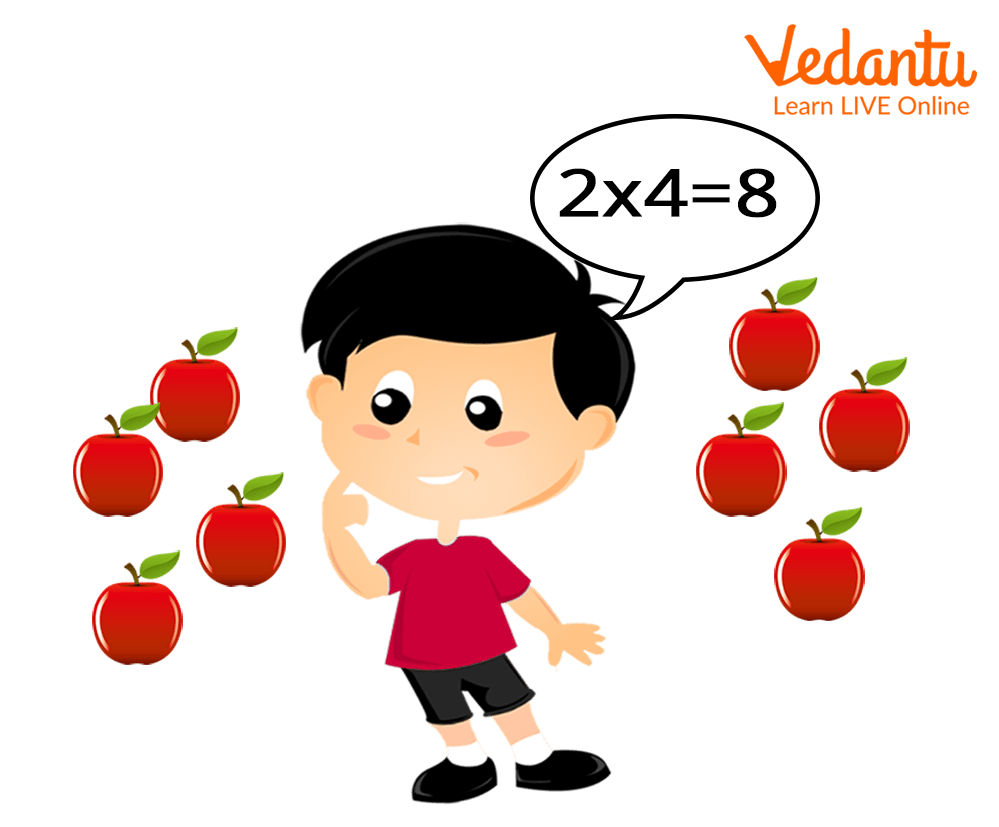Courses
Courses for Kids
Free study material
Free LIVE classes
More

# Teaching Multiplication to Kids in Grade 3LIVE
Join Vedantu’s FREE Mastercalss

## An Introduction to Multiplication in Year 3

As kids are promoted to the third grade, they are introduced to concepts like multiplication and division. Kids might know some basic rules and methods of the multiplication operation from the previous grades but it is important to have a strong understanding of the basics of multiplication.

The topics covered in multiplication in Grade 3 includes the multiplication of a two-digit number by a single digit. The article provides important topics that are taught in multiplication this year. The article also mentions some important methods that can help parents and teachers to develop a deeper understanding of the concept by the kids.

## Topics Taught in Multiplication for Kids

The topics taught in multiplication in year 3 include the simple multiplication process. The kids are introduced to the concept of multiplication. Kids are also encouraged to memorise tables of 2, 3, 4 and 5. Apart from multiplication, students are also introduced to simple division problems. These problems can be easily solved if a kid has a grasp of the table. A common technique that can be used to help students better understand multiplication is using real-life examples.

Example

A girl has 2 boxes of apples, each box has 4 apples. Find how many apples are there in total.

In this case, the total number of apples can be calculated by multiplying the number of apples by the number of boxes.

Total number of apples = 2 4= 8Multiplication of numbers

## Fun Ways of Teaching Multiplication in Grade 3

We now have a fair idea of some of the important topics that are covered in the syllabus of multiplication for kids in third grade. Let us look into some of the ways that can help parents and teachers to teach effectively.

### Practising Tables

Memorising tables is a critical skill in understanding the concepts of Mathematics. Parents can help kids in memorising the table by engaging with kids. Parents or teachers can print colourful cards that contain the number tables and learn them along with the kid.

### Using Real-life Examples

One of the most effective ways of teaching new concepts to kids is incorporating the concepts into the practical world. In the case of multiplication, parents can assist their children by demonstrating real-life instances of a timetable. A muffin tray, for example, will often feature four rows of three muffin cups apiece, demonstrating the multiplication 4 x 3.

### Using Different Strategies for Solving Multiplication Questions

At school, children will learn a variety of conceptual and informal strategies for solving multiplication questions, and some formal approaches such as column multiplication. Choose a multiplication problem and solve it along with your student and work out the answer independently to master these strategies.

Compare and contrast your approaches. Discuss which method is favoured, and why. This approach will help students to use the optimal approach for any given question since they will be able to employ various strategies. By allowing kids to practise a variety of approaches for multiplying numbers, you may help them acquire confidence in both formal and informal methods.

### Exploring Connections between Different Concepts

It is beneficial to be able to connect multiplication and division in order to solve complex mathematical problems. Encourage your kid to investigate the relationships between multiplication, arrays, and division, as well as the relationships between fractions and division. Although it is important to know that this can take a little more time as kids in third grade are newly introduced to the topic.

## Conclusion

This was the complete discussion on how to teach multiplication in Grade 3. We have explored the basic concepts that are taught in the topic; we have also looked into some of the methods that can help kids to develop a better understanding of the concept. An important point to note here is that it is through regular practice that a kid can enhance their skills. Lastly, we hope to have helped in providing insight on the introduction to multiplication.

Last updated date: 22nd Sep 2023
Total views: 134.4k
Views today: 1.34k

## FAQs on Teaching Multiplication to Kids in Grade 3

1. What is the array method in teaching multiplication?

The use of an array or column is a conventional approach in teaching multiplication to kids. In this method, a child is given a specific number of objects. They are then asked to arrange the objects in an array. After this, kids are asked to write as many calculations as they can using the array. For example, an array of 12 muffins (4 columns and 3 rows) can have the following calculations: 4 + 4 + 4 = 12, secondly kids can perform multiplication where 4 × 3 = 12. Kids can also be taught about division using the array. 12÷ 4 = 3.

2. What are some other topics covered in Mathematics apart from multiplication?

Addition, subtraction, simple division, use of the number system, classification of numbers, introduction to fractions, etc. are some of the important topics that are taught in Mathematics to kids in third grade. Understanding these topics helps kids excel academically as well helps in the development of understanding how things work in real life.